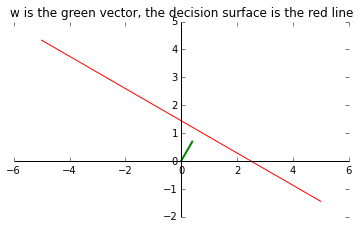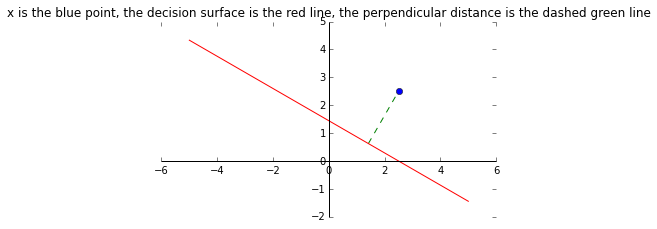In :
%pylab inline
def myshow(mytitle=None):
ax = axes()
ax.spines['left'].set_position('zero');
ax.spines['right'].set_color('none')
ax.spines['bottom'].set_position('zero');
ax.spines['top'].set_color('none')
ax.spines['left'].set_smart_bounds(True);
ax.spines['bottom'].set_smart_bounds(True)
ax.set_aspect('equal');
if mytitle is not None:
title(mytitle)
show()

Populating the interactive namespace from numpy and matplotlib

In :
# we see how the vector w define the orientation of the decision surface
# and how w_0 define the location of the decision surface
w_0 = -1.
w_length = .8
print("angle of the vector w: %.1f degrees"%degrees(w_angle))
print("normal distance from the origin to the deciison surface: %.1f"%(-w_0/w_length))
w = array([cos(w_angle), sin(w_angle)]) * w_length
print("w_1: %f w_2: %f"%(w,w))
x = linspace(-5,5,100)
# since w^Tx + w_0 = x1*w1 + x2*w2 + w_0 = 0 we can rearrange terms to get
# x2 = -(x1*w1 + w_0) / w2
x = column_stack((x, -(x*w + w_0)/w))
plot([0,w],[0,w], linewidth=2, color='g') # w vector
plot(x[:,0],x[:,1],'r') # decision surface
myshow("w is the green vector, the decision surface is the red line")

angle of the vector w: 60.0 degrees
normal distance from the origin to the deciison surface: 1.2
w_1: 0.400000 w_2: 0.692820In :
# we see how y(pointx) gives a signed measure of the perpendicular
# distance distancex of the point pointx from the decision surface
pointx = array([2.5,2.5])
distancex = (dot(w,pointx) + w_0) / w_length
print("perpendicular distance r of the point x from the decision surface: %f"%distancex)
# x_perp is found by rearraging terms in (4.6) on page 182
x_perp = pointx - distancex*(w/w_length)
plot([x_perp,pointx],[x_perp,pointx],'g', ls='dashed')
plot([pointx],[pointx],'bo') # arbitrary point x
plot(x[:,0],x[:,1],'r') # decision surface
myshow('x is the blue point, the decision surface is the red line, the perpendicular distance is the dashed green line')

perpendicular distance r of the point x from the decision surface: 2.165064